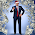## Monday, February 16, 2015

### MpRp=4LM Replaces e=mc^2 as Most Famous Equation!

Why does this simple equation work for the solution to the proton radius puzzle:

MpRp=4LM ???

Mp=Mass of proton
L=Planck Length
M=Planck Mass

Mp=1.67262177774e-27 kg
L=1.61619926e-33 cm
M=2.1765113e-8 kg

Calculated Rp=

Calculated Rp=0.84123643e-13 cm

The muonic hydrogen proton charge radius measurements of 2010 and 2013 approach this simply calculated value.

This is what Richard Feynman was looking for - a source of the mass of matter and it should be in fundamental units, Planck units, and that is what MpRp=4LM is.

(Nassim Haramein has solved this problem and resolved many of the unknown physics problems. It kinda goes without saying that what is interesting about this is the derivation of the solution...) See resonance.is and http://hiup.org/publications )
#science     #physics   #feynman     #nassimharamein   #bozon  ﻿

1.More soon. Developing...

2.Nassim'a theory not only explains this is replaces the Big Bang theory and explains SDSS J0100+2802

3.